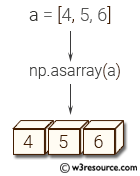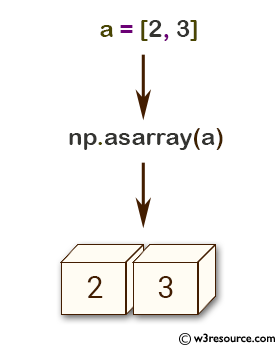# NumPy: numpy.asarray() function

## numpy.asarray() function

The numpy.asarray() function is used to convert a given input to an array.

This is useful when the input may be a list or a tuple, which cannot be used in array-specific operations.

Syntax:

`numpy.asarray(a, dtype=None, order=None)`Parameter:

Name Description Required /
Optional
a Input data, in any form that can be converted to an array. This includes lists, lists of tuples, tuples, tuples of tuples, tuples of lists and ndarrays. Required
dtype By default, the data-type is inferred from the input data. optional
order Whether to use row-major (C-style) or column-major (Fortran-style) memory representation. Defaults to 'C'. optional

Return value:

[ ndarray] Array interpretation of a. No copy is performed if the input is already an ndarray with matching dtype and order. If a is a subclass of ndarray, a base class ndarray is returned.

Example: np.asarray() function in NumPy

``````>>> import numpy as np
>>> a = [2, 3]
>>> np.asarray(a)
array([2, 3])
>>> x = np.array([2, 3])
>>> np.asarray(x) is x
True
``````

In the said code, the variable 'a' is a Python list containing two elements [2, 3]. When np.asarray(a) is executed, it creates a new NumPy array [2, 3]. Similarly, variable 'x' is a NumPy array [2, 3]. When np.asarray(x) is executed, it returns the original array 'x' because it is already a NumPy array.
The 'is' operator is used to check whether two variables refer to the same object in memory. In this case, np.asarray(x) is x returns True because 'x' is already a NumPy array and np.asarray() simply returns the original array without creating a new copy of it.

Pictorial Presentation:Example: Understanding np.asarray() function in NumPy

``````>>> import numpy as np
>>> x = np.array([2, 3], dtype=np.float32)
>>> np.asarray(x, dtype=np.float32) is x
True
>>> np.asarray(x, dtype=np.float64) is x
False
``````

In the above code the first line of code creates a NumPy array 'x' [2, 3] and then uses np.asarray() to convert it back into an array. Since 'x' is already an array, the np.asarray() function returns 'x'.
The second line of code converts the array 'x' to a float32 type array and checks whether the returned array is the same as 'x' using the 'is' keyword. Since 'x' is already a float32 type array, np.asarray() returns the same array 'x', so the condition is True.
The third line of code converts the array 'x' to a float64 type array and checks whether the returned array is the same as 'x' using the 'is' keyword. Since the data type of 'x' and the data type passed in np.asarray() is different, np.asarray() creates a new array with float64 data type and returns it, so the condition is False.

Example: Creating and converting structured arrays using NumPy

``````>>> import numpy as np
>>> issubclass(np.recarray, np.ndarray)
True
>>> a = np.array([(2.0, 3), (3.0, 5)], dtype='f4,i4').view(np.recarray)
>>> np.asarray(a) is a
False
>>> np.asanyarray(a) is a
True
``````

The first line of code checks if the np.recarray class is a subclass of the np.ndarray class, which it is.
The next line of code creates a structured array a with two fields, where the first field is a float32 and the second field is an int32. The view() method is used to convert the array to a record array.
The third line of code checks if np.asarray(a) and a refer to the same object, which is False since np.asarray(a) creates a new NumPy array object.
The final line of code checks if np.asanyarray(a) and a refer to the same object, which is True since np.asanyarray(a) returns a if a is already an array, otherwise it creates a new array object.

Python - NumPy Code Editor:

Previous: array()
Next: asanyarray()

﻿